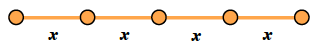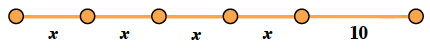### Home > MC1 > Chapter 5 > Lesson 5.1.1 > Problem5-6

5-6.

Four pieces of rope of unknown (and equal) length and $10$ more feet of rope are attached together. The resulting rope is $30$ feet long.

1. Draw a diagram to represent this problem.

• First, draw the $4$pieces of rope of unknown and equal length. A line segment with 4 equal sections, each labeled x.

• Then draw the $10$ foot rope. The line segment has an additional section added, labeled 10.

• Find a way to indicate that the whole rope is $30$ feet long.1. How long is each of the pieces of rope that is not $10$ feet? Show how you know.

• The four unknown lengths combine to be $20$ feet, so how long must each length be?